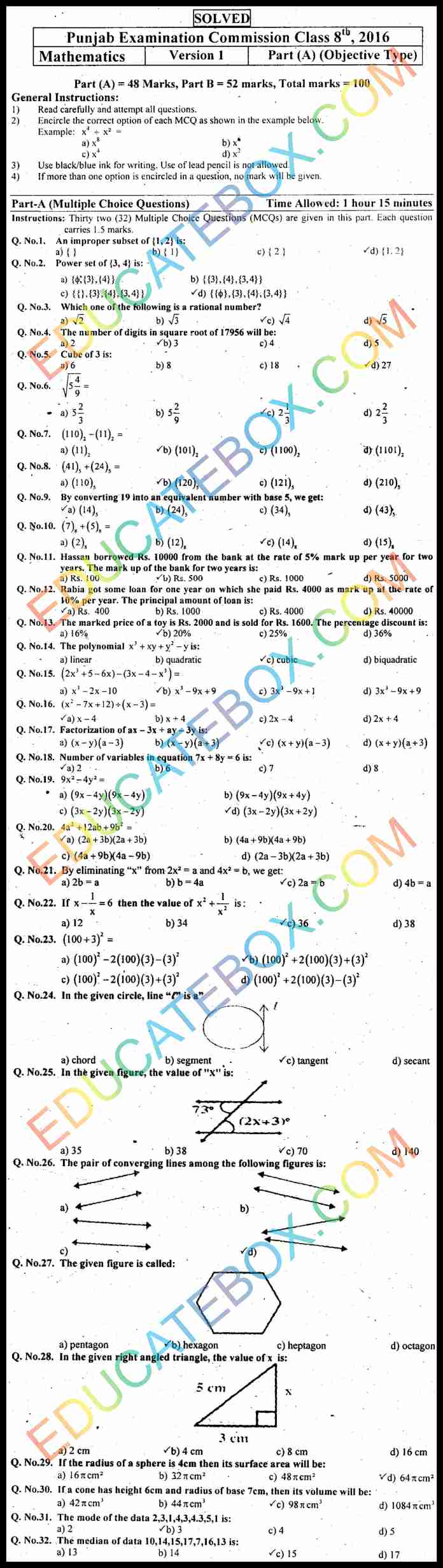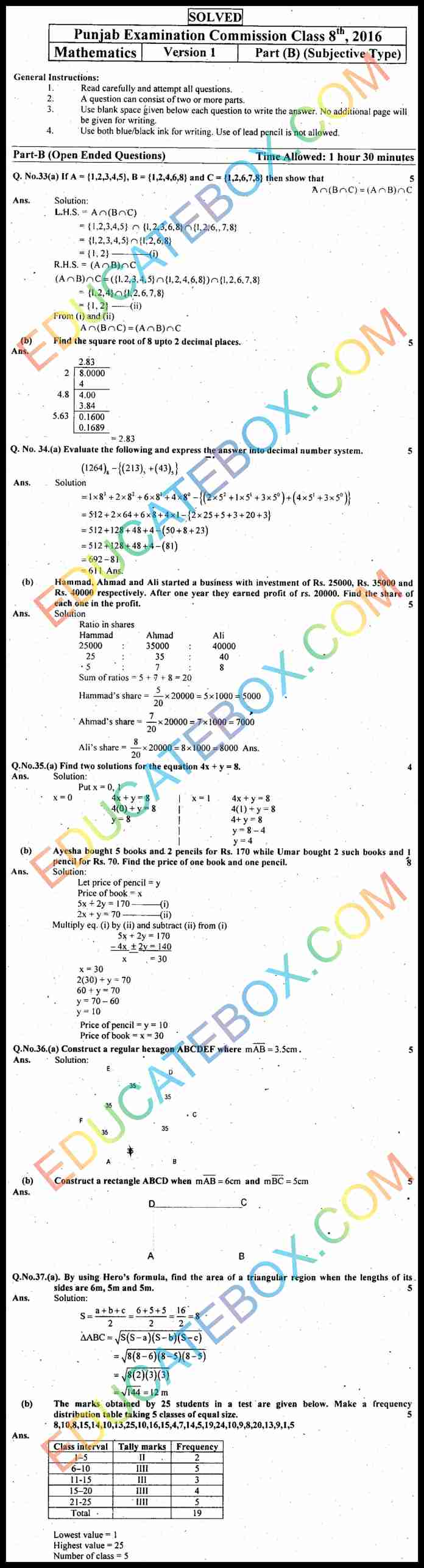# Past Paper 8th Class Maths 2016 Solved Paper EM Punjab Board (PEC) Version 1

1
1773

## Past Paper 8th Class Maths 2016 Solved Paper Punjab Board (PEC)  English Medium Version 1

8th class Punjab Examination Commission Maths exams held on Wednesday, February 17, 2016. This solved paper can be useful for the students.  This is for English medium students. Here is version 1 of this paper.  This is hundred marks paper.  Section A has 48 marks. Section B has 52 marks. Time allowed for first section is 1 hour and 15 minutes. While time allowed for Section B is 1 hour and 30 minutes.

Important questions asked in this paper include improper subset of {1,2}, power set of {3,4}, find  rational number from a set, number of digits in square root of 17956, cube of 3, binary division, binary minus, converting a number to base 5, polynomial, fraction of a number, number of variables in an equation, different types of graph, radius of sphere, mode of data element 2, 3, 1, 4, 3, 4, 3, 5, 1, and median of data 10, 14, 15, 17, 7, 16, 13. There are also some statement questions.

In the subjective first question is about Union and Intersection. Find out square root of  8 upto 2 decimal places. Evaluate the expression and express the answer into decimal number system. Find the solution of an equation. Using Hero’s Formula, find the area of triangle.

## اپ ٹو ڈیٹ پیپرایٹت کلاس میتھ سولڈ پیپر پنجاب بورڈ اوبجیکٹیو ٹائپ انگلش میڈیم ورژن 1## اپ ٹو ڈیٹ پیپرایٹت کلاس میتھ سولڈ پیپر پنجاب بورڈ اوبجیکٹیو ٹائپ انگلش میڈیم ورژن 1Save

#### 1 COMMENT

1.komal

very good